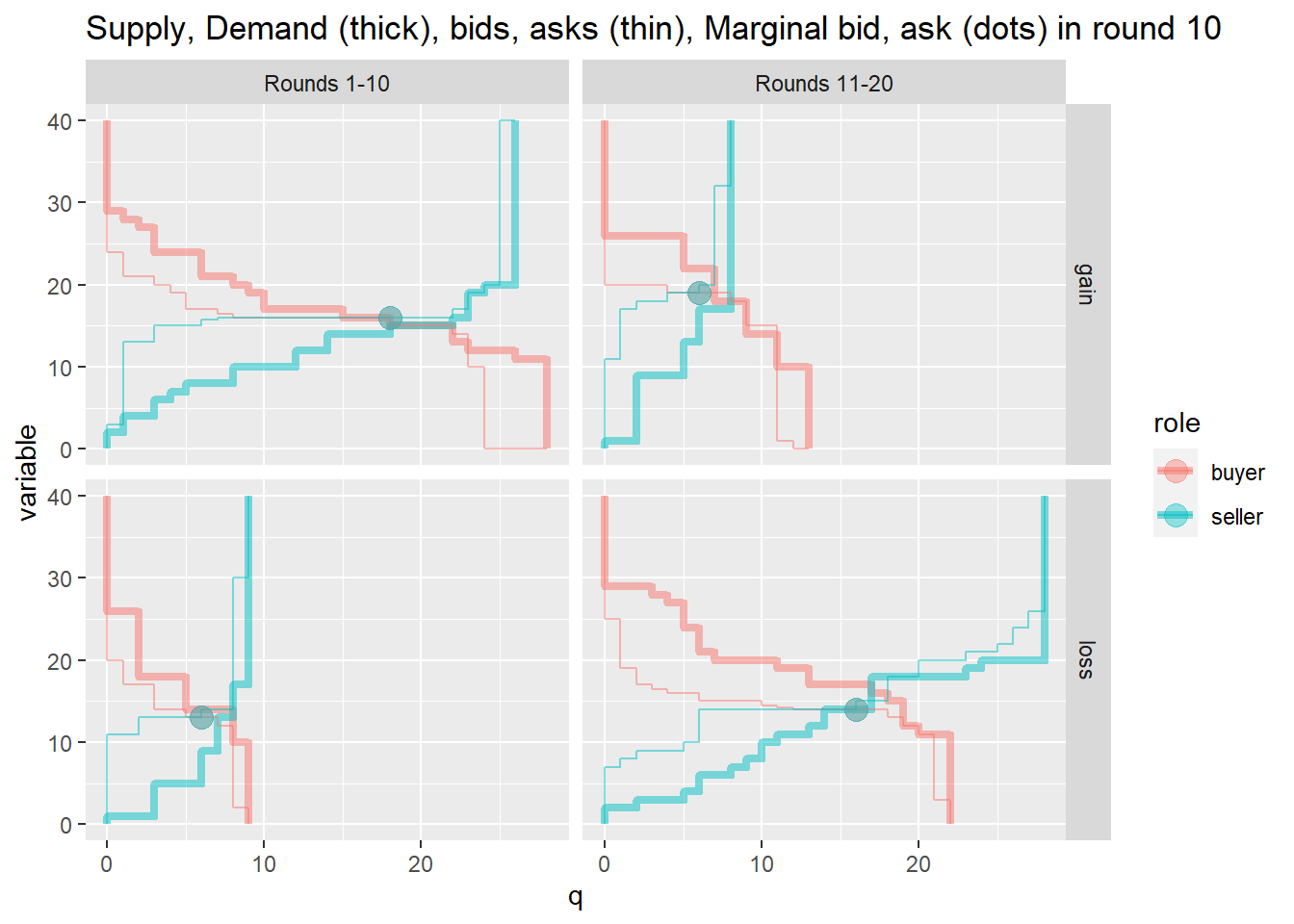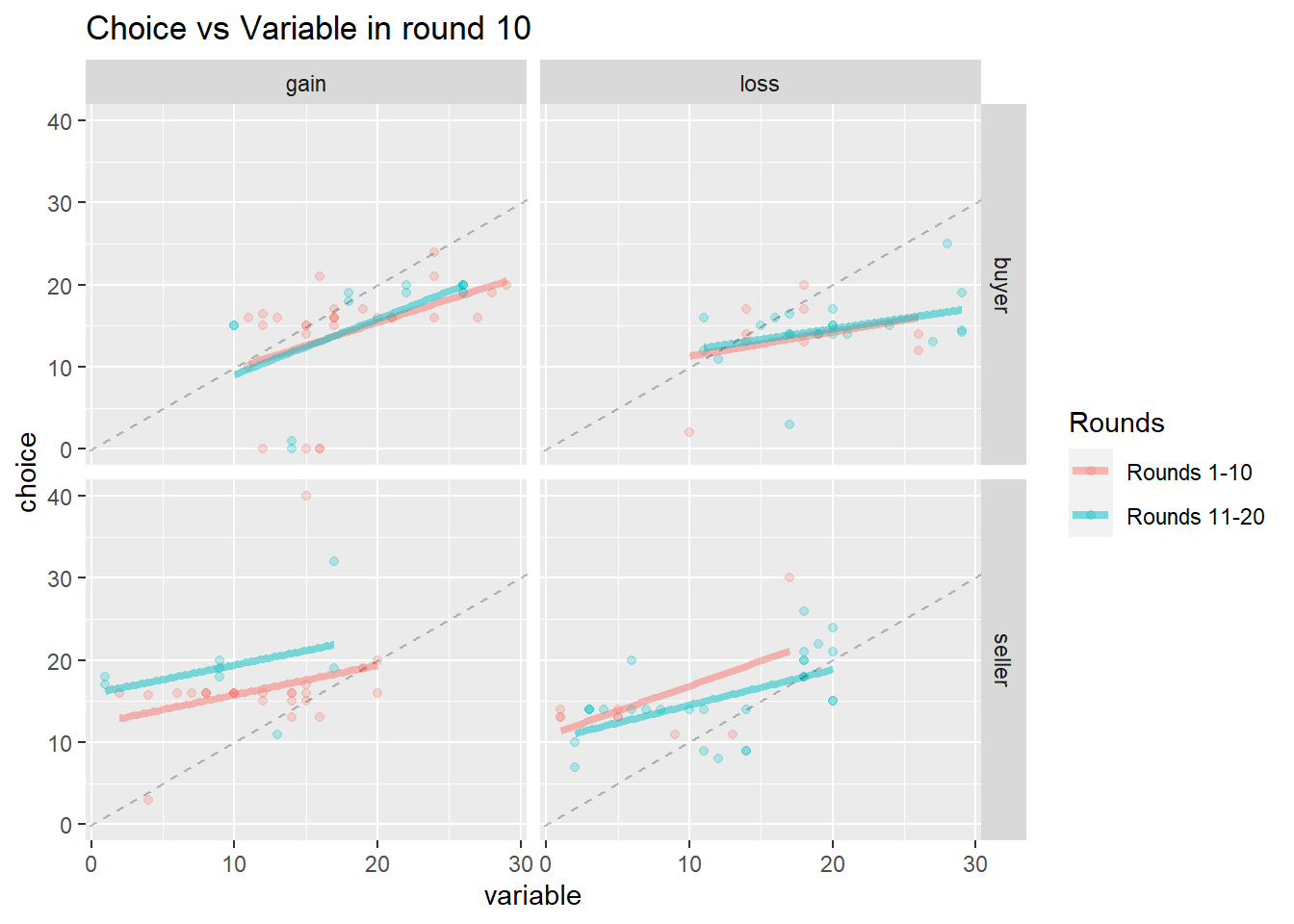Section # 01

Students must abide by UVic academic regulations and observe standards of scholarly integrity (i.e. no plagiarism or cheating). Therefore, this assignment must be taken individually and not with a friend, classmate, or group. You are also prohibited from sharing any information about the assignment with others. I affirm that I will not give or receive any aid on this assignment and that all work will be my own. N.Xiang

In environmental economics, perfectly competitive markets are our benchmark for efficiency: surplus is maximal under certain conditions:

• buyers and sellers take price as given.
• the market-maker knows the demand and supply curve (perfect information)
• there is no government intervention.
• there are no externalities.

The point of experiment 1 is to investigate how crucial are the assumptions of price taking and perfect knowledge. If the model of perfect competition does a good job of predicting behaviour even when these assumptions are not met, we can be more confident about using the model as our efficiency benchmark.

In experiment 1 the market-maker (in our case, the webserver) only makes use of the bids and asks when determining price, not the values and costs which are private information. Furthermore, your bid or ask may be the marginal bid or ask, determining either the price paid by demanders or the price received by suppliers. In a nutshell, we compare the predictions of perfect competition to the experimental outcomes to understand whether the assumptions of price taking and perfect information are substantive or merely for analytical convenience.

Recall that in experiment 1 buyers with bids weakly higher than the marginal bid purchase the good and suppliers with asks weakly lower than the marginal ask sell the good. We investigated two different market institutions regarding the prices paid and received. In one treatment the price paid by buyers was the marginal bid and the price received by sellers was the marginal ask: The difference between the marginal bid and marginal ask was the market maker’s gain per unit. In the other treatment the prices were reversed: the price paid by buyers was the marginal ask and the price received by sellers was the marginal bid. The difference between the marginal bid and marginal ask was the market maker’s loss per unit. The treatments were imbalanced in terms of the number of subjects: in rounds 1-10 most subjects were in the gain treatment, and then in rounds 11-20 most of the subjects were in the loss treatment. This allows us to look at the how the market institution (market-maker gain vs. loss) interacts with market depth.

# 1 (10 marks)

Suppose that you are a demander. If you are in the treatment where the market-maker makes a loss, could your bid ever determine the price buyers pay? If you are in the treatment where the market-maker makes a gain, could your bid ever determine the price buyers pay? How does market depth influence the probability your bid will determine the price buyers pay when the marketmaker gains?

# 2 (10 marks)

Create a function named salutations which takes as an argument person and prints a personalized greeting. The body of your function should be something like print(paste0("hello ",person,"!")). Hint: functions are defined like this function_name<-function(argument){body}. Functions are called like this: function_name("Rich")

#paste here your salutations function and call it using your name.

# 3 (0 marks)

Using dataframes supply_and_demand, bid_and_ask, and outcomes plot supply & demand, bids & asks and the marginal bid & ask for round_of_ten==10. Make the supply and demand prominent, and the bids and asks more subtle, and the marginal bid and ask as dots.# 4 (10 marks)

Explain what each line of code does to create first_plot. Hint: help on what built-in functions do can by found by typing ?x in the console, where x is the name of the function. Suppose that you were interested in looking at all the rounds in round_of_ten, not just round 10. What would you modify in the code for first_plot for each different round? How many lines of code would you have if you cut/pasted/modified the code that created first_plot to create second_plot, third_plot… tenth_plot?

# 5 (10 marks)

Use the code that created first_plot to write a function plot_sd() that takes as an argument rnd_of_10. The body of plot_sd() will contain the code that created first_plot, except wherever there was a 10 you need to replace it with rnd_of_10. Hint: you can test the function by typing plot_sd(10) and it should look identical to first_plot. Use assert_that to make sure the input to your function is valid: i.e. rnd_of_10 %in% 1:10. How many lines of code would you have in total for the function, and 10 calls to that function?

#paste a copy of your plot_sd function in this code chunk.

# 6 (10 marks)

Use your function plot_sd() to plot the supply, demand bids and asks in round 10.

# 7 (10 marks)

Copy and paste the code that created first_plot, and rename it third_plot. Add the aesthetic frame=round_of_ten inside the aes(). i.e mapping=aes(q,variable, colour=role, frame=round_of_ten). Remove the filtering of the data used by both the geom_step() calls e.g instead of data=filter(supply_and_demand,round_of_ten==10) use data=supply_and_demand. Run the code to create third_plot by hitting control plus enter but do not look at at the plot. Instead, if third_plot ran without error, copy the following code into your assignment1.R file and hit control plus enter. How many lines of code were required to see the plots for all 10 rounds?

(third_plot <- ggplotly(third_plot)%>%
animation_opts(1000,transition = 100)%>%
hide_legend()%>%
config(displayModeBar = F))

Insert your animation (third_plot) below:

# 8 (10 marks)

Describe the dataframe mydf. You can view the dataframe by clicking on it in the top right panel of Rstudio, and it will open in the top left panel. What are the columns, and what are the rows?

# 9 (10 marks)

Explain what the following code snippet does. Hints: <- is the assignment function (give the result on the right the name on the left). %>% is the pipe function, read as THEN (take what is on the left THEN apply the function on the next line). For functions that have alphabetic names you can type ?name in the console to get help. e.g. ?group_by

value_and_cost <- mydf%>%
group_by(marketmaker,round_of_ten, Rounds, role, variable)%>%
count(variable)

# 10 (10 marks)

Cut and paste the above code snippet into your assignment1.R file and save. Source the assignment1.R and click on value_and_cost in the top right panel. How can dataframe value_and_cost be used to check to make sure that function plot_sd is plotting the supply and demand correctly?

# 11 (0 marks)

For round_of_ten==10, plot the relationship between choice and variable for all combinations of marketmaker and role, using a different colour for Rounds# 12 (10 marks)

Copy and paste the code that created fourth_plot, and rename it fifth_plot. Add the aesthetic frame=round_of_ten inside the aes(). i.e mapping=aes(variable,choice,frame=round_of_ten, colour=Rounds). Remove the filtering of the data e.g. instead of data=filter(mydf, round_of_ten==10) use data=mydf. Run the code to create fifth_plot by hitting control plus enter but do not look at at the plot. Instead, if fifth_plot ran without error, copy the following code into your assignment1.R file and hit control plus enter.

(fifth_plot <- ggplotly(fifth_plot)%>%
animation_opts(1000,transition = 100)%>%
hide_legend()%>%
config(displayModeBar = F))